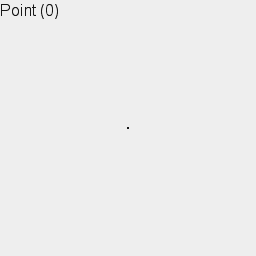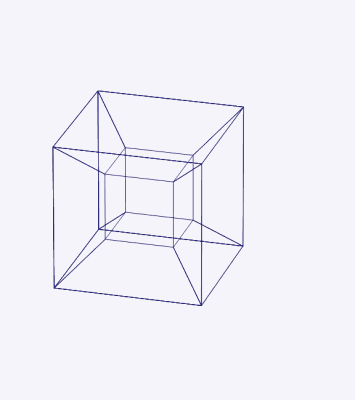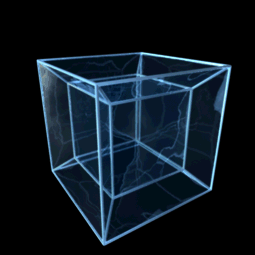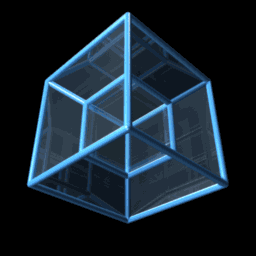# What Is A Tesseract? A 4D Object That’s Impossible To Build

• A tesseract is a four-dimensional hypercube with 24 faces, 32 edges, and 16 vertices.
• It can be created by thickening up a cube in a fourth dimension.
• Although it is impossible to physically construct a tesseract, we can visualize it in our 3D world.

The idea of the fourth spatial dimension has tantalized people since its conception. In physics, the three dimensions represent space (x,y,z) while the fourth dimension represents time (t). However, in an abstract mathematical concept, there could be an infinite number of spatial dimensions.

Let’s try to understand the fourth dimension. In geometry, the four-dimensional analogue of a cube is called tesseract. It is easy to extrapolate by looking at lower dimensions –

• Zero-dimensional cube is a point, a vertex
• One-dimensional cube is a line segment with 2 vertices (one at each end). It can be created by thickening up a point in one dimension.
• Two-dimensional cube is a square with 4 vertices. It can be created by thickening up the line segment in the second dimension.
• Three-dimensional cube is a cube with 8 vertices, created by thickening up the square in a third dimension.

Similarly, a four-dimensional cube (also known as hypercube or tesseract) has 16 vertices. It can be created by thickening up a cube in a fourth dimension. But since we live in a 3D world, it’s impossible to construct a 4D object.

Overall, you can say that a tesseract is to the cube as the cube is to the square. It has 24 faces, 32 edges, and 16 vertices.An object shifting in dimensions, from Point to Tesseract | Credit: Wikimedia Commons

### Tesseract Is Very Hard To Visualize

It is extremely difficult if not impossible to visualize tesseract or any other four-dimensional object. This is because our imaginations are not strong enough to project our consciousness into an artificial world that is very different from our own.

Our brains are wired to convert two-dimensional input into a three-dimensional view. More specifically, our eyes send a pair of two-dimensional image to the brain, from which the brain constructs a 2D+depth model of the visual field.

This is what our brains are best suited for thinking about. Three-dimensional space is easy to visualize because we literally see it all the time. However, we have no direct experience of higher dimensions, and thus people have no clear prototype to use as a springboard for visualizing it.

Reference: What is a four-dimensional space like? | John D. Norton

Physicists and mathematicians trained in higher-dimensional-spaces works, one the other hand, are more capable than the rest of us to visualize it in their brain.

Let’s Try To Visualize A Tesseract

Like a cube can be projected into two-dimensional space, it is possible to project tesseracts into three-dimensional space.Figure 2 | Image credit: Wikimedia Commons

The surface of a 3D cube contains 6 square faces, similarly, the hypersurface of the tesseract contains 8 cubical cells.

One can unfold a tesseract into 8 cubes into three-dimensional space (figure 2). This is similar to unfolding a cube into 6 square into two-dimensional space. An unfolding of a geometric object [with flat sides] is known as a net. The tesseract features 261 different nets.

There are two types of four-dimensional rotations:

1) Simple Rotations: A three-dimensional projection of the Tesseract (figure 3) performing a simple rotation about a plane bisecting figure from top to bottom and front-left to back-right.Figure 3 | Alternative projection of tesseract | Wikimedia Commons

2) Double Rotation: A three-dimensional projection of the tesseract (figure 4) showing a double rotation about 2 orthogonal planes.Figure 4 | Alternative projection of tesseract | Wikimedia Commons

The tesseract can also be shown from a hidden volume elimination perspective. In figure 5, for instance, the red face is closest to the fourth dimension and has four cubical cells meeting around it.Figure 5 | Tesseract from hidden volume elimination perspective

### It Was Discovered In 1888

The word “tesseract” was coined by a British mathematician and science fiction writer Charles Howard Hinton. He used the word for the first time in 1888 in his book ‘A New Era of Thought‘. He also invented several new words to describe elements in the fourth dimension.

Since then the word “tesseract” has been used in different kinds of art, architecture and science fiction stories (such as Avengers and Agents of S.H.I.E.L.D), where it has nothing to do with the four-dimensional hypercube.Written by
###### Varun Kumar

Varun Kumar is a professional science and technology journalist and a big fan of AI, machines, and space exploration. He received a Master's degree in computer science from Indraprastha University. To find out about his latest projects, feel free to directly email him at [email protected]